# 2nd PUC Physics Previous Year Question Paper June 2017

Students can Download 2nd PUC Physics Previous Year Question Paper June 2017, Karnataka 2nd PUC Physics Model Question Papers with Answers helps you to revise the complete Karnataka State Board Syllabus and score more marks in your examinations.

## Karnataka 2nd PUC Physics Previous Year Question Paper June 2017

Time: 3 Hrs 15 Min
Max. Marks: 70

Instructions:

1. All parts are compulsory.
2. Answer without relevant diagram/figure/circuit wherever necessary will not carry any marks.
3. Numerical problems solved without writing the relevant formulae carry no marks.

Part – A

I. Answer ALL the following questions: (10 × 1 = 10)

Question 1.
How does the electrostatic force between two point charges change, when a dielectric medium is introduced between them?
Electrostatic force between the two point charges decreases when a dielectric medium is placed between them.

Question 2.
State Kirchhoff’s junction rule.
Kirchhoff’s junction rule states that the algebraic sum of currents entering and leaving the mode of an electrical network is zero.Question 3.
What is Lorentz force?
The combined electric and magnetic force on a moving charge is called Lorentz force.

Question 4.
Write the relation connecting rms value and peak value of alternating current.
Irms = $$\frac{I_{p e a k}}{\sqrt{2}}$$ = 0.7071peak

Question 5.
What is motional electromotive force?
The amount of induced e.m.f across a moving conductor in a uniform magnetic field is called motional e.m.f.

Question 6.
Mention the value of power factor of a pure capacitor.
The power factor of a pure capacitor is zero.

Question 7.
Define half life of a radioactive sample.
The time for 50% of the radioactive sample to decay is called half life of the element.

Question 8.
Give the circuit symbol of AND-gate.
Circuit symbols of AND-gate:Question 9.
Write any one advantage of light emitting diode.
The LED can be operated at a very low DC voltage. The switch on time is very low and efficiency is very high.

Question 10.
What is attenuation in communication system?
The fall in the strength of the signal over distance is called attenuation.

Part – B

II. Answer any Five of the following questions: (5 × 2 = 10)

Question 11.
State and explain Gauss’s law in electrostatics.
Statement:
‘The total outward Electric flux in air through any closed surface is equal to (1/ε0) times the total charge enclosed by it’. Let q1, q2, q3 ………….. qn be the charge enclosed by a system of discrete charges.
According to gauss theorem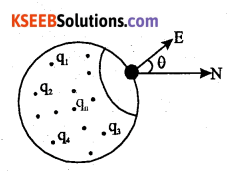Φ = E cos θ (∑ds) where
‘∑ds’ is the effective area through which component of Electric field ‘E cos θ’ does not vanish.

Question 12.
Define mobility. Mention its S.I. Unit
Electron mobility is defined as the ratio of drift velocity of electron to the applied electric field. The S.I.U of electron mobility is m2V-1s-1.Question 13.
What is ohmic device? Give one example.
Ohmic devices are those devices which obey Ohm’s law. Conductor at room temperature.

Question 14.
Write any two differences between diamagnetic and paramagnetic substances.

 Diamagnetic substances Paramagnetic substances 1. These substances are repelled by a strong magnetic field. These are slightly attracted towards a strong magnetic field. 2. These substances move from a stronger to a weaker magnetic field. These substances move from a weaker to a stronger magnetic field. 3. 0 ≤ μr < 1 0 ≤ μr < 1 + ∈ where ∈ is a small positive integer. 4. ‘χ’ is low but negative and is of the order -10-9 to -10-6 ‘χ’  is low but positive and is of the +10-6 to +10-4 5. Susceptibility is independent of temperature. χ α  $$\frac{1}{\mathrm{T}}$$ where ‘T’ is the absolute temperature of the substance.

Question 15.
The magnetic flux linked with a coil varies as Φ = 3t2 + 4t + 9. Find the magnitude of the emf induced at t = 2S.
Induced emf = $$-\frac{d \phi}{d t}$$
= $$-\frac{d}{d t}$$(3t2 + 4t + 9)
= -(6t + 4) volt
Induced emf at t = 2 ,
e = -(6 × 2 + 4)
= -16 V.

Question 16.
Write Maxwell’s equation for the speed of electromagnetic waves and explain the terms.
Speed of light in vacuum c = $$\frac{1}{\sqrt{\mu_{0} \varepsilon_{0}}}$$
Where m0 is absolute magneticQuestion 17.
What are de-Broglie Waves? How does the de-Broglie wavelength vary with momentum of moving particle?
de Broglie equation is λ = $$\frac{h}{m v}$$ where λ is the de Broglie wavelength, m is the mass and v is the velocity of the particle. de-Broglie wavelength ‘λ’ is inversely proportional to the linear momentum of the particle i.e, λ α $$\frac{1}{\mathrm{MV}}$$ where linear momentum p = mv

Question 18.
Draw the block diagram of generalised communication system.
Block diagram of generalised communication system:Part – C

III. Answer any Five of the following questions: (5 × 3 = 15)

Question 19.
Obtain the relation between electric field and electric potential due to a point charge.
Consider a point charge ‘+q’. Let ‘P’ be a point initially at infinity. Let ‘A’ be a point inside the . field region. Let ‘+1C’ be a unit positive charge moved from the point at infinity to point ‘A’. Let ‘A’ be at a distance of ‘r’ from the given point charge. Let ‘B’ be another point at distance of ‘dr’, from’A’towards the point charge +q.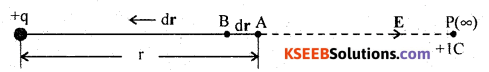The work done in moving positive test charge from A to B, against the force of repulsion is dW = -Fdr. The -ve sign indicates that the work is done against the direction of the force and dr is the displacement of +1C of test charge in the direction opposite to the electric field.
But electric potential dV = $$\frac{d W}{+q_{0}}$$ and electric field E = $$\frac{\mathrm{F}}{+q_{0}}$$
When q0 = +1C then dW = dV, E = F
Hence dV = -E dr or E = $$-\frac{d \mathrm{V}}{d r}$$
i.e., Electric field intensity at a point is the negative potential gradient at that point and electric field intensity is in the direction of decreasing electrostatic potential.Question 20.
Derive the relation $$\overrightarrow{\boldsymbol{j}}=\sigma \overrightarrow{\mathbf{E}}$$ with terms which has usual meaning.
We know from the Ohm’s law, I = KV
where, I – electric current, K – conductance and V – potential difference
Dividing I = KV by ’A’ on both sides we get,
$$\frac{I}{A}=\frac{K}{A} \cdot V$$
by definition electric current density j = $$\frac{I}{A}$$Hence j = $$\left(\frac{\mathrm{K}}{A}\right)(E l)$$.
where σ = $$\frac{\mathrm{K} l}{A}$$ is known as the electrical conductivity.
Hence j = σ E. In a vector form, the relation is written as $$\vec{j}=\sigma \vec{E}$$

Question 21.
How can a moving coil galvanometer be converted into a voltmeter? Explain with a diagram.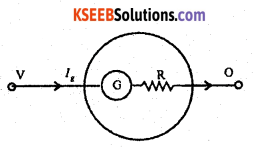To convert a galvanometer into a voltmeter, a high resistance is connected in series with the coil. The galvanometer with this modification is called a voltmeter. The value of the high resistance R to be connected in series with the coil depends on the maximum potential difference to be measured. If Ig be the current for a full scale deflection then,
V = Ig(G + R)
G is the resistance of the galvanometer.
R = $$\frac{V}{I_{g}}$$ – G
Ig ∝ V
Since G and R are constants, the scale can be graduated to read potential differences directly. A voltmeter is used for measurement of potential difference. It should be connected in parallel in a circuit.Question 22.
Mention any three applications of eddy currents.
The circulating currents set up in bulk pieces of metal through a magnetic field are called eddy currents.
Practical applications of eddy currents:

1. Magnetic brakes work on the principle of eddy currents. When a magnetic field is applied on the rotating drum, eddy currents are induced in the drum. The eddy currents decrease steadily in magnitude as the train slows down. Thus the braking effect is quite smooth.

2. Energy meters are instruments used to measure the electrical energy consumed by the electrical appliances. A metal disc in the energy meter rotates due to eddy currents.

Other applications:

3. Induction (ac) motor (rotor) is a low resistance coil placed in a rotating magnetic field. Eddy currents in a rotor, rotates it in a direction of the magnetic field.

4. Eddy currents of high frequencies are used in the induction furnace which is used to, prepare alloys by melting the constituent metals.

Question 23.
Define critical angle. Write two conditions for total internal reflection.
Consider a point object in the denser medium. There will be cone of rays striking the interface. For a ray of light OA, it suffers normal refraction. For any angle of incidence less than critical angle such as ray OB, the refracted ray bends away from the normal.

However for a particular angle of incidence called critical angle, the refracted ray of light grazes the interface. Therefore critical angle for the given pair of media and given wavelength of light may be defined as the angle of incidence in the denser medium for which the refracted ray is at right angles to the normal drawn at the point of incidence.For a ray of light such as OD, whose angle of incidence is greater than the critical angle, there will be no refraction of light, instead the light bounces back into the same denser medium. This phenomenon of light is known as the total internal reflection.
Conditions for the total internal reflection:

1. Object should be in the denser medium and viewed through rarer medium.
2. Angle of incidence in the denser medium should be more than the critical angle for the given pair of media and wavelength of light.Question 24.
Write any three differences between interference and diffraction.

 Interference Diffraction 1. Two or more waves travelling in a forward direction when superposed, form an interference pattern. Light bends around the corners of a sharp edge and encroach the region of shadow of an obstacle. 2. Two independent and coherent waves superpose to form interference pattern. Secondary waves emerging from a single wave front result in diffraction pattern. 3. Interference fringes are of uniform intensity. All bright fringes are equally bright. Central maximum has maximum width and subsequent secondary maxima have diminishing intensity. 4. There is a good contrast between maxima and minima. Comparatively a poor contrast exists between maxima and minima. 5. The condition for Cl is nλ, = d sin where n = 0, 1, 2, 3, ……….. The condition for Cl is (2n + 1)$$\frac{\lambda}{2}$$ = d sin θ where n = 1,2, 3, ……….. 6. The condition for D1 is (2n + 1) $$\frac{\lambda}{2}$$ = d sin θ The condition for DI is nλ = d sin θ, n = 1, 2,3,…

Question 25.
Define the terms:

1. Threshold frequency
2. Work function.
3. Stopping potential.

1. Threshold frequency is the minimum frequency of the incident radiation below which photo emission cannot take place in the substance.
2. The minimum energy which is just enough to liberate electrons from a given surface is called its photo electric work function.
3. The minimum negative potential of the anode for which all photo electrons are prevented from reaching the anode is called the stopping potential. Stopping potential is directly proportional to the frequency above the threshold frequency.

Question 26.
Explain the use Zener diode as a voltage regulator.
Circuit diagram:The circuit connections are made as shown in the figure. The circuit contains a zener diode, an unregulated voltage source (Vin > VZ), a series resistance RS and a load resistance RL.

Any increase/decrease of voltage drop across RS produce no change in voltage across the zener diode. For (Vin < VZ), the zener diode does not function. Thus the zener diode acts as voltage regulator.Part – D

IV. Answer any Two of the following questions: (2 × 5 = 10)

Question 27.
Derive an expression for the electric field due to an electric dipole at a point on the axial line.
Let +1C be ihe test charge at P, at a distance r from the centre of an electric dipole. Test charge + 1C experiences a force of repulsion along BP due to +q at B and attraction due to -q along PA.

Since ‘+q’ is nearer than -q from P, the resultant force points along BP. Force experienced by a unit +ve charge is called electric field.
Net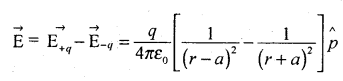where, $$\hat{p}$$ represents the unit vector of dipole moment point from – q to + q.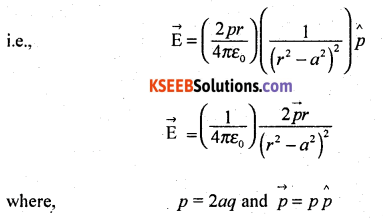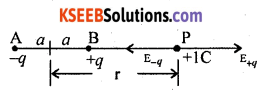Note:Question 28.
Obtain an expression for the force between two straight parallel conductors carrying current. Hence define ampere.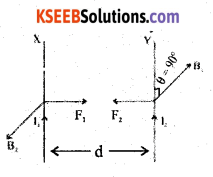X and Y are two long straight parallel conductors carrying currents I1 and I2 respectively, and placed close to each other. d is the separation between the two conductors and L is the length of the conductors.

The magnetic field at any point on the conductor Y due to current I1 in the conductor X, is given byActs in a direction perpendicular to the plane containing the two conductors. The conductor Y which carries current I2, experiences a mechanical force due to Bx acting on it and this force is given by F1 = B1 I2 L sin θ.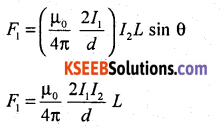According to Fleming’s left hand rule, the direction of the force F1 on X is perpendicular to B2 and is towards the conductor Y. The magnetic field at any point on the conductor X due to the current I2 in y, is given byActs on X and opposite to B1. The mechanical force acts on X due to B2 is I1 L sin θ.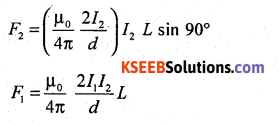According to Fleming’s left hand side rule, the direction of the force F2 on X is perpendicular to X and it is towards the conductor y if the current I1 is inwards (or away from the conductor Y if the current I1, is outwards)

The Force F1 acting on a certain length of the conductor Y due to the current in the conductor X is equal in magnitude to the force F2 acting on the same length of X due to the current in conductor Y.

If the two conductors carry the currents in the same direction (parallel currents) then the forces attract each other. If the two conductors carry the currents in the opposite directions (anti parallel currents), then they are found to repel each other because the two forces act away from each other.
The force per unit length on each conductor is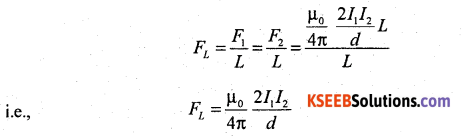One ampere of current can be defined as that constant current which when maintained through each of the two infinitely long straight parallel conductors of negligible area of cross section in the same direction placed 1 metre apart in vacuum, causes an attractive force of 2 × 10-7 N m-1 length on each conductor.

Question 29.
Show that a current carrying solenoid is equivalent to a bar magnet.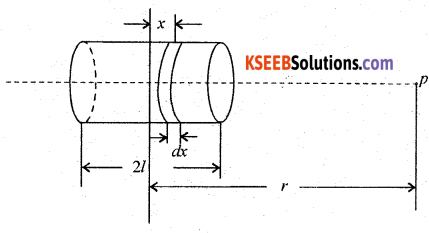The magnitude of magnetic field at a point ‘P’ due to a circular element of thickness ‘dx’ is given by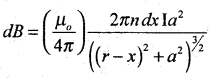The magnitude of total magnetic field is given byWe note that the product n(2l) I (πa2) = Magnetic moment ‘m’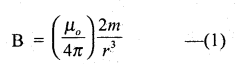This expression is similar to the magnetic field at a point on the axis of a short magnet. Therefore a bar magnet and a solenoid produce similar magnetic fields.V. Answer any Two of the following questions: (2 × 5 = 10)

Question 30.
Derive the lens maker’s formula.
Lens maker’s formula:Let r1 and r2 be the radii of curvature of the thin lens. Let O be the point object at a distance u from the pole of the first curved surface of the lens. Real image is formed at I’, at a distance v’ from the pole P1. This image is formed in the denser medium.
We know that from the refraction formula,where n1 is the refractive index of rarer medium and n2 that of the denser medium (n2 > n1). For the second surface, real image at I will serve as a virtual object and the distance is taken as -ve. The object space is the lens medium for refraction through the second curved surface. Final image is formed in air at I and at a distance of V from P2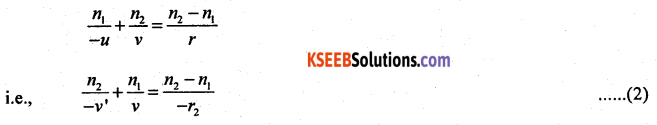Adding (1) and (2) we getwhere u = ∞, v = f
when u = f, v = ∞
∴ The term on the L.H.S. can be replaced by $$\frac{1}{f}$$ where f is the focal length of lens.The equation (4) is called the lens maker’s formula.
Note:
(1) Using the equation (4), it can be shown that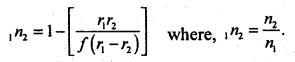(2) For radius of curvature the letter ‘R’ may be used.

Question 31.
Derive an expression for the total energy of an electron in stationary state of hydrogen atom. Assuming the expression for the radius.
Consider an electron of mass m and charge -e revolving round the nucleus of an atom of atomic number Z in the nth orbit of radius ‘r’. Let v be the velocity of the electron. The electron possesses potential energy, because, it is in the electrostatic field of the nucleus. The electron also possesses kinetic energy by virtue of its motion.

Potential energy of the electron is given by,
Ep = (potential at a distance r from the nucleus) (-e)Kinetic energy of the electron is given by,
Ek = $$\frac{1}{2}$$ mv2 _______(2)
From Bohr’s postulate,Substituting this value of mv2 in equation (2)
Ek = $$\frac{1}{2}\left(\frac{Z e^{2}}{4 \pi \varepsilon_{0} r}\right)$$ ____(3)
Total energy of the electron revolving in the orbit is given by En = Ep + Ek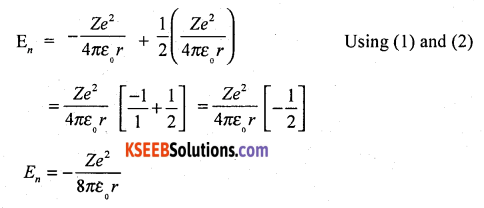The radius of nth permitted orbit of the electron is given by r = $$\frac{\varepsilon_{0} n^{2} h^{2}}{\pi m Z e^{2}}$$. Substituting this value of r in the equation,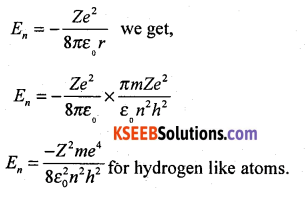For hydrogen atom, put Z = 1
∴ Total energy of the electron in the orbit of hydrogen atom is En = $$\frac{-m e^{4}}{8 \varepsilon_{0}^{2} n^{2} h^{2}}$$
Note: The total energy of the electron in the first orbit is E1 = $$\frac{-m e^{4}}{8 \varepsilon_{0}^{2} h^{2}}$$ = – 13.6 ev.

Question 32.
What is amplification? With a circuit diagram, explain the working of npn transistor as an amplifier in CE configuration.
A small change in base current produces a large variation in the collector current. This property of an amplifier is known as amplification.
1. Collector end is reverse biased and base – emitter ends forward – biased.

2. A small variation in the base current produces a large variation in the collector current with a phase difference of 180° between them, without altering the input frequency. This is the basis of an amplifier circuit.

3. Power gain of the amplifier in CE mode is given by,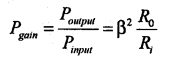Where, current gain β = $$\frac{I_{0}}{I_{1}}=\frac{I_{C}}{I_{B}}$$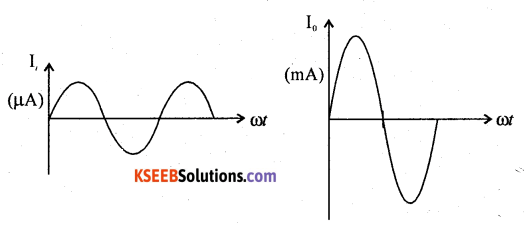4. The input and out put currents are as shown in the figure above.VI. Answer any three of the following questions: (3 × 5 = 15)

Question 33.
In a parallel plate capacitor with air between the plates , each plate has an area 8 × 10-3 m2 and distance between the plates is 2 mm. Calculate the capacitance of the capacitor. If this capacitor is connected to a 50 V supply, what is the charge on each plate of the capacitor? (Absolute permittivity of free space = 8.85 × 10-12 Fm-1)
Given A = 8 × 10-3 m2
d = 2 × 10-3 m
Cair = ?
V = 50V,Q = ?, e0 = 8. 85 × 10-12 Fm-1
WKT electrical capacitance of an air capacitor
C = $$\frac{\varepsilon_{0} A}{d}$$
i.e; C = $$\frac{8.85 \times 10^{-2} \times 8 \times 10^{-3}}{2 \times 10^{-3}}$$
i.e, C = 35.416 × 10-2 F(farad)
Also, WKT Q = charge an the capacitor = CV
i.e, Q = 35.416 × 10-12 × 50
= 1770.8 × 10-12 C
Q= 1.771 × 10-9 C (coulomb)Question 34.

1. Three resistors 4 W, 6 W, and 8 W, are combined in parallel. What is the total resistance of the combination?
2. If the combination is connected to a battery of emf 25 V and negligible Internal resistance, then determine the current through each resistor and total current drawn from the battery.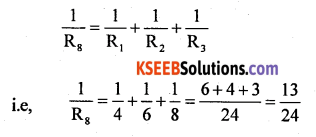Given R1 = 4W; R2 = 6W; R3 = 8W;
1. WKT, for parallel combination,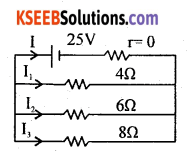Hence equivalant resistance in parallel conbination is Rp = $$\frac{24}{13}$$ = 1.846Ω2. Main current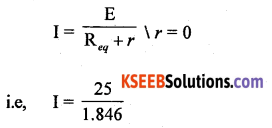I = 13.543 A
Total current drawn from the battery is 13.543 A
Branch current I1= $$\frac{25}{4}$$ = 6.250 A
I2= $$\frac{25}{6}$$ = 4.168 A
& I3= $$\frac{25}{8}$$ = 3.125 AQuestion 35.
A sinusoidal voltage of peak value 285 V is applied to a series LCR circuit in which resistor of resistance 5 W, pure Inductor of Inductance 28.5 mH and capacitor of capacitance 800 μ F are connected.

1. Find the resonant frequency.
2. Calculate the impedance, current and power dissipated at the resonance.

Given V0 = 285 V; R= 5W; L = 28.5 × 10-3 H ;
C = 800 × 106 F.
1. Resonant frequency f0 = $$\frac{1}{2 \pi \sqrt{\mathrm{LC}}}$$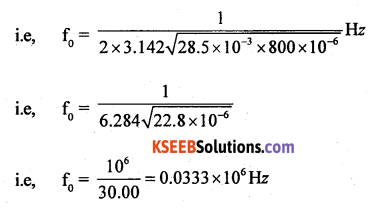i.e, f0 = 33.3 Hz

2. At resonance XL = XC and impedance Z = R.
Hence impedance = 5W; I = $$\frac{V}{Z}$$
i.e; Current Ipeak = $$\frac{\mathrm{V}_{\text {peak}}}{5}=\frac{285}{5}$$ = 57 A.
Power dissipated = $$\frac{V_{p} I_{p}}{2}$$ cosΦ
Where f = 0° & cos f = cos 0° = 1 at resonance.
Power dissipated = $$\frac{285 \times 57}{2}$$ = 812.5 W .
= 8.123 kW (kilowatt)Question 36.
In Young’s double slit experiment distance between the slits is 0.5 mm. When the screen is kept at a distance of 100 cm from the slits, the distance of ninth bright fringe from the centre of the’fringe system is 8.835 mm. Find the wavelength of light used.
Given: d = 0.5 mm = 5 × 10-4 m
D = 100cm = 1 m
x = 8.835 × 10-3 m., n = 9
l = ?
WKT, the distance of Bright fringe from the Central bright fringe is given by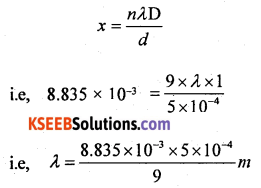ie, λ = 4.0983 × 10-7 m
or λ = 4.098.3 A°Question 37.
Calculate the Binding energy and binding energy per nucleon of an oxygen nucleus (816O) using the following data(MeV):
Mass of proton = 1.007825 u
Mass of neutron = 1.008665 u
Mass of oxygen nucleus = 15.995 u.
Given: Mp = 1.007825 u
Mn = 1.008665 u
Mox = 15.995 u
Rert Mass of Constituent Nucleus:
Mass of 8 protons = 8 × 1.007825 = 8.0626 u
Mass of 8 neutrons = 8 × 1.008665 = 8069324
total mass = 8.0262+ 8.6932 = 16.13194
rest mass of 816O nucleon = 15.99504
Mass defect of the Nucleon = 0.13694
Energy equivalent of mass defect = 0.1369 × 9.31.5 MeV
B.E= 127.522 MeV
$$\frac{B \cdot E}{A}=\frac{127.522}{16}$$ = 7.970 MeV/nucleon.
Hence B.E.per nucleon = 7.970 MeV/nucleon.

error: Content is protected !!